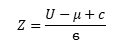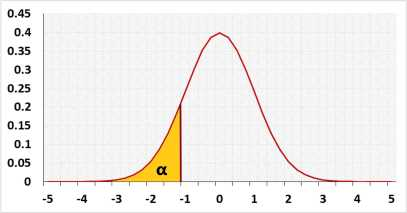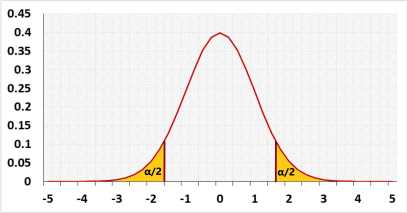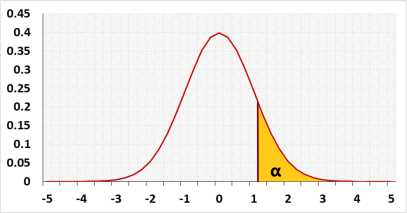# Mann Whitney U test calculator (Wilcoxon rank-sum)

Non-parametric test
The test compares the probability to get higher value from group1 with the probability to get higher value from group2.

## Test calculation

Enter raw data directly
Enter raw data from excel

## Enter sample data

Header: You may change groups' name to the real names.
Data: When entering data, press Enter, , or Space after each value.

 Group1 Group2

The tool ignores empty cells or non-numeric cells.

## Enter sample data

You may copy data from Excel, Google sheets or any tool that separate data with Tab and Line Feed.
Copy the data, one block of 3 consecutive columns includes the header, and paste below.
Copy the data,It is okay to leave empty cells, empty cells or non numeric cells won't be counted

### Results

Skewness Shape:

The difference between the expected SD and the sample SD

validation message

## Information

Hypotheses
H0: Group1 = Group2
H1: Group1 < > Group2
Test statisticNormal distributionC - continuity correction, when U > μ: C = -0.5, when U < μ: C = 0.5

### Target

Unlike t-test that compares the means, the Mann-Whitney U test compares a randomly selected value from group1 to a randomly selected value from group2.
When the two distributions have a similar shape you can use the test to compare also the medians.
When the two distributions have a similar symmetrical shape, you can use the test to compare also the means. more

### Method

The mann whitney u test calculator may use three methods. We reccomend to use the "Automatic" method.
Automatic - when n1≤20 and n2≤20 and the data doesn't have ties, the tool uses the exact value, otherwise the tool uses the z approximation.
Exact - when n1≤20 and n2≤20 the tool uses the exact value, calculated base on all the possible combinations, otherwise the tool uses the z approximation.
Z approximation - the tool uses the z approximation.

Continuity correction - relevant only for the Z approximation. It is used since a continuous distribution is used to calculate a discrete distribution.

## R Code

The following R code should produce similar results: